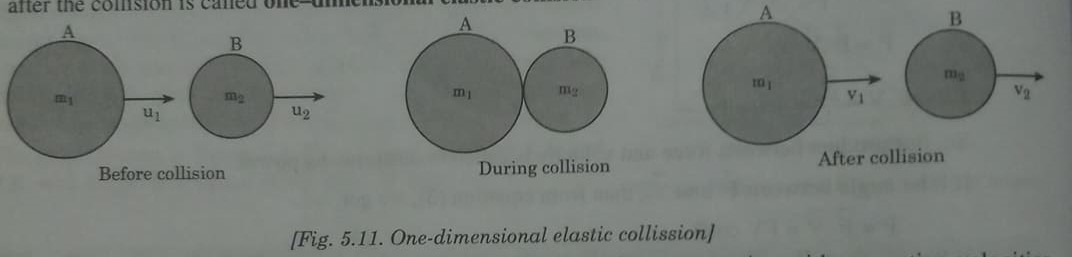Elastic collision in One DimensionThe elastic collision in which the colliding particles moving along the same straight line path before and after the collision is called one-dimensional elastic collision.Consider two bodies A and B having respective masses m1 and m2 are moving with respective velocities u1 and u2 is straight line such that u1 > u2 before collision as shown in figure . Let, the bodies collide elastically and v1 and v2 be their respective velocities after collision.

Applying law of conservation of linear momentum,

m1u1 + m2u2 = m1v1 +m2v2                         ..........(1)

or,       m1(u1 – v1)   = m2(v2 – u2)                          ...........(2)

Since in an elastic collision, total kinetic energy also remains conserved. Therefore,From equation (7) and (8), the velocities of the bodies A and B, after collision can be calculated.

Special cases

·        When both the colliding are of the equal masses then, m1 = m2 = m(say)

From(7), v1 = u2

From(8), v2 = u1

This shows that if two bodies having equal masses collide elastically in one-dimension, then they simply interchange their velocities after the collision.